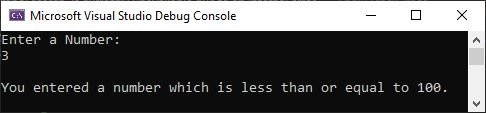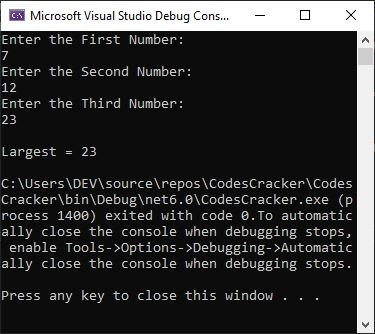# C# if else | C# Conditional Statements

In this article, we will discuss conditional statements in C#. Conditional statements are used to tell the program what to do based on certain conditions. The list of conditional statements we will discuss in this section is as follows:

## C# if statement

The if statement is used when we need to execute some defined block of code if the specified condition evaluates to be true. For example,

```Console.WriteLine("Enter a Number: ");

if (num <= 100)
Console.WriteLine("\nYou entered a number which is less than or equal to 100.");```

This C# example of the if statement (with user input 3) should give the exact following result:Now what if the user enters a number greater than 100?
I mean, there is no block of code to be executed if the given condition evaluates to false. So for this solution, we have if...else 😄. Let's discuss it.

## C# if...else statement

The if...else statement is similar to the if statement. The only difference is that we will have another block of code that will be executed if the specified condition evaluates to be false. For example:

```Console.WriteLine("Enter a Number: ");

if (num <= 100)
Console.WriteLine("\nYou entered a number which is less than or equal to 100.");
else
Console.WriteLine("\nYou entered a number which is greater than 100.");```

Now, with user input 234, the output should exactly be:

```Enter a Number:
234

You entered a number which is greater than 100.```

Now we have another problem. The problem is, what if we need to test multiple conditions?
The solution is that we have if...else if...else statement 😆. Let's discuss it.

## C# if...else if...else Statement

The general form of the if...else if...else statement in C# is:

```if (condition_1)
{
// block of code to be executed
// if condition_1 evaluates to be true
}
else if (condition_2)
{
// block of code to be executed
// if condition_2 evaluates to be true
}
else if (condition_3)
{
// block of code to be executed
// if condition_3 evaluates to be true
}
else if (condition_4)
{
// block of code to be executed
// if condition_4 evaluates to be true
}
.
.
.
else if (condition_N)
{
// block of code to be executed
// if condition_N evaluates to be true
}
else
{
// block of code to be executed
// if all specified condition evaluates
// to be false
}```

Please note: If any condition from all the conditions, evaluates to be true, then the rest all the conditions will be skipped. Here is an example demonstrating the if...else if...else statement in C#.

```int num = -45;
if (num > 0)
Console.WriteLine("Positive number");
else if (num == 0)
Console.WriteLine("Zero");
else
Console.WriteLine("Negative Number");```

Since both of the above conditions should be false, the code inside the else block will be run. Therefore, the output should exactly be:

`Negative Number`

We can also nest one if inside another, or one if...else inside another, and so on. I think I need to take another example of a nested if...else if...else statement before completing this section.

```Console.WriteLine("Enter any Number: ");

if (num > 0)
{
if (num > 10)
Console.WriteLine("It is greater than 10.");
else if (num > 100)
{
if (num > 50)
Console.WriteLine("It is greater than 50.");
else
Console.WriteLine("It is greater than 100.");
}
else if (num > 500)
Console.WriteLine("It is greater than 500.");
else if (num > 1000000)
Console.WriteLine("It is greater than 1000000.");
else
Console.WriteLine("It is less than 10.");
}
else if (num == 0)
Console.WriteLine("It is 0.");
else
{
if (num > -10)
Console.WriteLine("It is between -1 to -9.");
else
Console.WriteLine("It is less than -9.");
}```

The output with user input 5 should be:

```Enter any Number:
5
It is less than 10.```

Please note: It is not required to use {} braces, if the block has a single line of code. Therefore, in above C# example, the if, else, and else...if block containing a single line of code was not wrapped in curly braces.

Please note: To do the job of if...else in a single line of code, or to create shortcut code of if...else block, we have the ternary operator (? :) 😁. The general form of ternary operator in C# is:

`var = (condition) ? x : y;`

The value of x should be initialized to var if the condition evaluates to be true. Otherwise, the value of y should be initialized to var. Now let's understand how we can implement it in a C# program.

```int a = 10, b = 20, large;
large = (a > b) ? a : b;

Console.WriteLine("Largest = {0}", large);```

The output should exactly be:

`Largest = 20`

since the value of a is not greater than b. Therefore, b (20) was initialized to large. Let me give you another example, which you need to fully understand how the ternary operator works in C#.

```Console.WriteLine("Enter the First Number: ");

Console.WriteLine("Enter the Second Number: ");

Console.WriteLine("Enter the Third Number: ");

int large = (a > b) ? ((b > c) ? a : (c > a) ? c : a) : (b > c) ? b : c;

Console.WriteLine("\nLargest = {0}", large);```

The snapshot given below was taken after executing the above C# example (with user inputs of 7, 12, and 23), demonstrating the ternary operator in C# by finding the largest of three numbers through it:In the above example, in the following code:

`(a > b) ? ((b > c) ? a : (c > a) ? c : a) : (b > c) ? b : c`

First, we have the condition part, which is: (a > b). Then we have two expressions, the first being "expression 1" and the second being "expression 2". The expression 1 is ((b > c) ? a : (c > a) ? c : a), which will be evaluated if the defined condition evaluates to be true. Otherwise the expression 2, which is (b > c) ? b : c, that will be executed if the defined condition evaluates to be false.

Now since the condition a > b or 7 > 12 evaluates to be false. Therefore, (b > c) ? b : c replaced the whole expression. Because b > c or 12 > 23 evaluates to be false, therefore the value of c which is 23 will be the result of the whole expression and will be initialized to the large variable.

C# Online Test

« Previous Tutorial Next Tutorial »